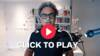# How to Use AI & ChatGPT To Grow Your eCommerce Leads, Sales & Revenue In 7 Days...``` // Set the target time (23 minutes and 7 seconds from now) let targetTime = new Date(); targetTime.setMinutes(targetTime.getMinutes() + 0); targetTime.setSeconds(targetTime.getSeconds() + 3); // Get the timer elements const hoursElement = document.getElementById("countdown-hours"); const minutesElement = document.getElementById("countdown-minutes"); const secondsElement = document.getElementById("countdown-seconds"); // Update the timer every second const countdownInterval = setInterval(() => { // Calculate the remaining time let now = new Date(); let remainingTime = Math.max(targetTime - now, 0); // Calculate hours, minutes, and seconds let hours = Math.floor(remainingTime / 3600000); let minutes = Math.floor((remainingTime % 3600000) / 60000); let seconds = Math.floor((remainingTime % 60000) / 1000); // Update the timer elements hoursElement.textContent = hours.toString().padStart(2, "0"); minutesElement.textContent = minutes.toString().padStart(2, "0"); secondsElement.textContent = seconds.toString().padStart(2, "0"); // If the timer has reached zero, stop the interval and replace the timer with a button if (remainingTime === 0) { clearInterval(countdownInterval); document.getElementById("countdown-content").style.display = "none"; const button = document.createElement("a"); button.textContent = "Join eCommerce Growth Machine Today"; button.classList.add("button"); button.href = "https://ecombrew.co/join"; button.rel = "noopener noreferrer"; // add this line document.getElementById("button-container").appendChild(button); } }, 1000); ```